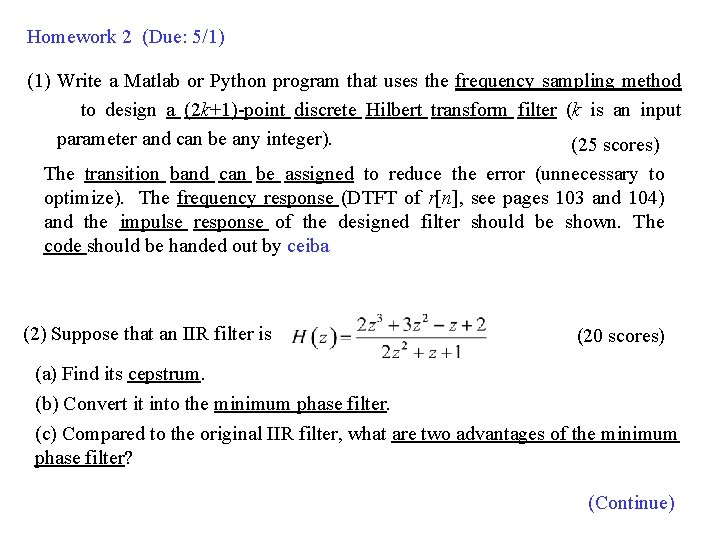# Homework 2 Due 51 1 Write a Matlab

• Slides: 3
Download presentationHomework 2 (Due: 5/1) (1) Write a Matlab or Python program that uses the frequency sampling method to design a (2 k+1)-point discrete Hilbert transform filter (k is an input parameter and can be any integer). (25 scores) The transition band can be assigned to reduce the error (unnecessary to optimize). The frequency response (DTFT of r[n], see pages 103 and 104) and the impulse response of the designed filter should be shown. The code should be handed out by ceiba (2) Suppose that an IIR filter is (20 scores) (a) Find its cepstrum. (b) Convert it into the minimum phase filter. (c) Compared to the original IIR filter, what are two advantages of the minimum phase filter? (Continue)(3) Suppose that x[n] = 1+cos (2 n). (a) What is the Hilbert transform of x[n]? (b) What is the analytic function corresponding to x[n]? (10 scores) (4) Among the following filters: (i) the Notch filter (ii) the difference, (iii) the Hilbert transform, (iv) the Kalman filter, (v) the Wiener filter, and (vi) the particle filer, (a) Which filters are suitable for edge detection? (b) Which filters are suitable for prediction? (10 scores) (5) Suppose that How do we use the cepstrum and the lifter to recover x[n] from y[n]? (10 scores) (6) (a) Why the cepstrum is more suitable for solving the multi-path problem than the equalizer? (describe at least 2 reasons) (b) Why the Mel-frequency cepstrum is more suitable for dealing with the acoustic signal than the original cepstrum? (describe at least 3 reasons) (15 scores) (Continue)(7) Suppose that there are three vocal signals: (i) sin(400 t), (ii) cos(1200 t), (iii) sin(3600 t). (a) Which voice sounds louder? (b) Which voice signal can be propagated in a longer distance? (10 scores) (Extra): 根據你的學號來回答 4/10, 4/17, 4/24 上課影片中的問題 (共兩題)